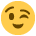#### Howdy, Stranger!

It looks like you're new here. If you want to get involved, click one of these buttons!

Supported by

# How to collect the age of a subject as a variable

Hi,
when starting an experiment, I would like to enter subject ID, date of birth and current date and then have opensesame log the sub_ID and sub_age for every trial/response as a variable. Ideally I would like to log both age in days and years.

I found information on getting the days between dates in python (https://stackoverflow.com/questions/151199/how-do-i-calculate-number-of-days-between-two-dates-using-python) but since I'm new to both OS and python, I'd like to learn from an example solution.

• Hi,

I recommend to use `text_input_forms`. You can collect all kinds of information like that.

The error message seems to be quite straightforward, it

Good luck,

Eduard

• edited May 2018

Hi there, i was looking for the same thing.
And probably it would help everyone if there was a python code (based on text_input_form) which is accessible to everyone. So everybody could just paste it into an inline_script and that's it.

It should do the following:
#1 ask for Birthdate in the format DD.MM.JJJJ
#2 calculate diff between experiment_date and Birthday
#3 log the exact age in years (with commas)

Up to now i was not able to manage that.
But maybe @eduard this is an easy thing to do?Cheers,
Stephan

• Thanks for this masterpiece http://osdoc.cogsci.nl/3.2/manual/forms/validation/

I changed the code a little to get the exact age with Birthday.
Here is the code for anyone who is interested``````def my_form_validator():

"""Checks whether both the gender and age fields have been filled out"""
#also checks for the number of characters
return var.sex != u'no' and var.byear != u'' and var.bmonth != u'' and var.bday != u'' and len(str(var.byear))==4 and len(str(var.bmonth))==(1 or 2) and len(str(var.bday))==(1 or 2)

def filter_digits(ch):

"""Allows only digit characters as input"""

return ch in ['0','1','2','3','4','5','6','7','8','9','backspace']

# Define all widgets
button_ok = Button(text=u'Ok')
checkbox_male = Checkbox(text=u'Male', group=u'gender', var=u'sex')
checkbox_female = Checkbox(text=u'Female', group=u'gender', var=u'sex')
label_year = Label(u'Year')
label_month = Label(u'Month')
label_day = Label(u'Day')
# Specify a key filter so that only digits are accepted as text input
input_year  = TextInput(stub=u'2018 …', var=u'byear',  key_filter=filter_digits) #actually a selection thing would be nice
input_month = TextInput(stub=u'01 …',   var=u'bmonth', key_filter=filter_digits)
input_day   = TextInput(stub=u'01 …',   var=u'bday',   key_filter=filter_digits)

# Build the form. Specify a validator function to make sure that the form is
# completed.
my_form = Form( validator=my_form_validator, cols=3, rows=6, spacing=10, margins=(100, 100, 100, 100),  )
#my_form.set_widget(label_gender, (0, 0))
my_form.set_widget(checkbox_male, (1, 0))
my_form.set_widget(checkbox_female, (2, 0))
my_form.set_widget(label_age, (1, 1))
my_form.set_widget(label_year, (0, 2))
my_form.set_widget(input_year,  (1, 2), colspan=2)
my_form.set_widget(label_month, (0, 3))
my_form.set_widget(input_month, (1, 3), colspan=2)
my_form.set_widget(label_day, (0, 4))
my_form.set_widget(input_day,   (1, 4), colspan=2)
my_form.set_widget(button_ok, (1, 5))
my_form._exec()

# Calculate age from current time and Birtdate
import shutil,os,datetime

now = datetime.datetime.now()
current_year  = now.strftime("%Y")
current_month = now.strftime("%m")
current_day   = now.strftime("%d")
#current_time = now.strftime("%Y-%m-%d")

years  = int(current_year) - var.byear
months = int(current_month) - var.bmonth
days   = int(current_day) - var.bday

var.age= years + float(months)/12 + float(days)/365
var.Bday = str(var.byear)+'.'+str(var.bmonth)+'.'+str(var.bday)
print var.age, var.Bday
log.write_vars()
``````
• And for those who need an ID_code
Info: use Bday and ID_code
a) to check whether a participants participates twice in the same experiment
b) or whether the same participant participated in two of your experiments

``````def ID_code_validator():

"""Checks whether all fields have been filled out with one character"""
#also checks for the number of characters
return var.X1 != u'no' and var.X2 != u'' and var.X3 != u'' and var.X4 != u'' and len(str(var.X1))==1 and len(str(var.X2))==1 and len(str(var.X3))==1 and len(str(var.X4))==1

ALPHA = []
for i in range(65,91):
ALPHA.append(chr(i))
alpha = []
for i in range(97,123):
alpha.append(chr(i))
list_alphabet = ALPHA + alpha + ['backspace']

def filter_alphabet(ch):

"""Allows only alphabetic characters as input"""

return ch in list_alphabet

# Define all widgets
button_ok = Button(text=u'Ok')

# Specify a key filter so that only digits are accepted as text input
input_father  = TextInput(stub=u'X …', var=u'X1', key_filter=filter_alphabet)
input_mother  = TextInput(stub=u'X …', var=u'X2', key_filter=filter_alphabet)
input_own     = TextInput(stub=u'X …', var=u'X3', key_filter=filter_alphabet)
input_bplace  = TextInput(stub=u'X …', var=u'X4', key_filter=filter_alphabet)

# Build the form. Specify a validator function to make sure that the form is
# completed.
my_form = Form( validator=ID_code_validator, cols=4, rows=10, spacing=10, margins=(100, 100, 100, 100), )
#my_form.set_widget(label_gender, (0, 0))
my_form.set_widget(label_father, (0, 0), colspan=3)
my_form.set_widget(input_father, (3, 0))
my_form.set_widget(label_mother, (0, 2), colspan=3)
my_form.set_widget(input_mother, (3, 2))
my_form.set_widget(label_own,    (0, 4), colspan=3)
my_form.set_widget(input_own,    (3, 4))
my_form.set_widget(label_bplace, (0, 6), colspan=3)
my_form.set_widget(input_bplace, (3, 6))
my_form.set_widget(button_ok,    (3, 8))
my_form._exec()

var.ID_code= var.X1 + var.X2 + var.X3 + var.X4
print 'ID_CODE:', var.ID_code
log.write_vars()
``````
• Nice!

Is there a way to change the fontsize?

Thanks,

Sylvain

• I always work with these standard settings in the "general options":
Foreground black
Background white
Font mono 18px

With that it looks nice.

• Apparently changing fontsize is doable:
http://forum.cogsci.nl/index.php?p=/discussion/507/solved-change-backgrounds-colors-and-fonts-in-widgets

But for some reasons I struggle to do it, for instance:

``````text_OK = "<span font-size = 12 >OK</span>"
button_ok = Button(text=text_OK)
``````

doesnt seem to work.